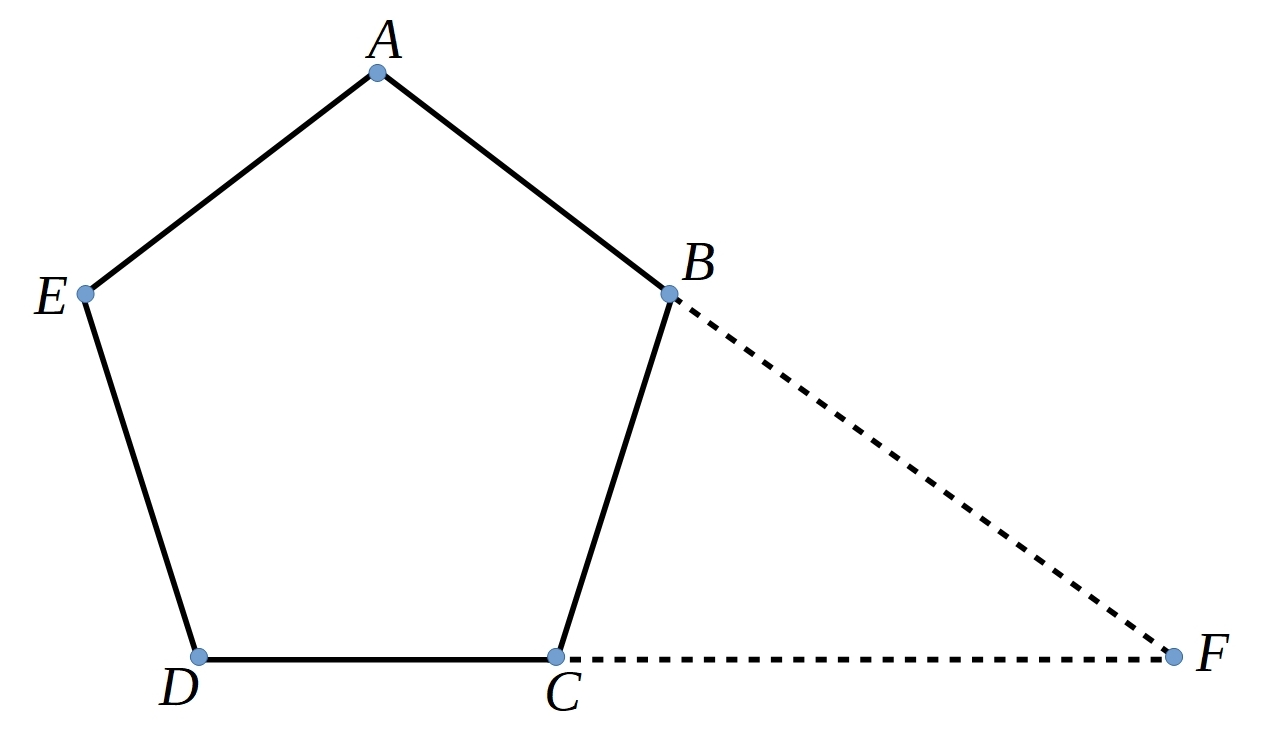#### You may also like### At a Glance

The area of a regular pentagon looks about twice as a big as the pentangle star drawn within it. Is it?### Golden Triangle

Three triangles ABC, CBD and ABD (where D is a point on AC) are all isosceles. Find all the angles. Prove that the ratio of AB to BC is equal to the golden ratio.### Darts and Kites

Explore the geometry of these dart and kite shapes!

# Pentakite

##### Age 14 to 18Challenge Level$ABCDE$ is a regular pentagon of side length one unit.

The lines $AB$ and $DC$ are extended until they meet at another point, $F$.

Can you show that triangle $BFC$ is congrugent to triangle $DAC$?

Can you find a pair of similar triangles?

Can you use these results to find the length $DA$?

You can read more about the Golden Ratio in this Plus Article.

We are very grateful to the Heilbronn Institute for Mathematical Research for their generous support for the development of this resource.